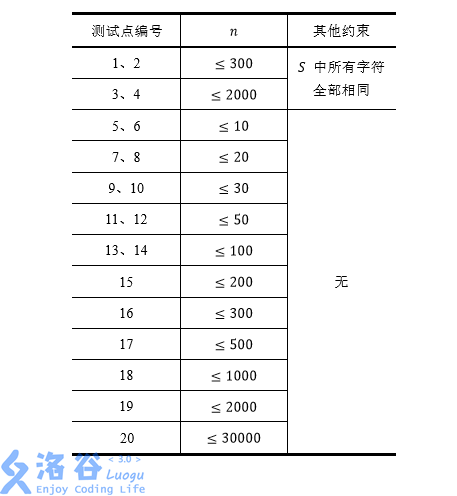# P1117 [NOI2016]优秀的拆分

• 1.3K通过
• 3.7K提交
• 题目提供者
• 评测方式 云端评测
• 标签 后缀数组,SA 字符串 枚举,暴力 NOI系列 2016 O2优化 高性能
• 难度 NOI/NOI+/CTSC
• 时空限制 1000ms / 128MB
• 提示：收藏到任务计划后，可在首页查看。

## 题目描述

如果一个字符串可以被拆分为$AABB$的形式，其中 A和 B是任意非空字符串，则我们称该字符串的这种拆分是优秀的。

例如，对于字符串$aabaabaa$，如果令 $A=aab$，$B=a$，我们就找到了这个字符串拆分成 $AABB$的一种方式。

一个字符串可能没有优秀的拆分，也可能存在不止一种优秀的拆分。比如我们令 $A=a$，$B=baa$，也可以用 $AABB$表示出上述字符串；但是，字符串 $abaabaa$ 就没有优秀的拆分。

现在给出一个长度为 $n$的字符串$S$，我们需要求出，在它所有子串的所有拆分方式中，优秀拆分的总个数。这里的子串是指字符串中连续的一段。

以下事项需要注意：

1. 出现在不同位置的相同子串，我们认为是不同的子串，它们的优秀拆分均会被记入答案。

2. 在一个拆分中，允许出现$A=B$。例如 $cccc$ 存在拆分$A=B=c$。

3. 字符串本身也是它的一个子串。

## 输入输出格式

输入格式：

每个输入文件包含多组数据。

输入的第一行只有一个整数$T$，表示数据的组数。保证 $1≤T≤10$。

接下来 $T$行，每行包含一个仅由英文小写字母构成的字符串$S$，意义如题所述。

输出格式：

输出 $T$行，每行包含一个整数，表示字符串$S$ 所有子串的所有拆分中，总共有多少个是优秀的拆分。

## 输入输出样例

输入样例#1： 复制
4
aabbbb
cccccc
aabaabaabaa
bbaabaababaaba

输出样例#1： 复制
3
5
4
7


## 说明

我们用$S_{i,j}$表示字符串 $S$第 $i$个字符到第$j$个字符的子串（从$1$开始计数）。

第一组数据中，共有 $3$个子串存在优秀的拆分：

$S_{1,4}=aabb$，优秀的拆分为$A=a$，$B=b$；

$S_{3,6}=bbbb$，优秀的拆分为 $A=b$，$B=b$；

$S_{1,6}=aabbbb$，优秀的拆分为 $A=a$，$B=bb$。

而剩下的子串不存在优秀的拆分，所以第一组数据的答案是 $3$。

第二组数据中，有两类，总共$4$个子串存在优秀的拆分：

对于子串 $S_{1,4}=S_{2,5}=S_{3,6}=cccc$，它们优秀的拆分相同，均为$A=c$，$B=c$，但由于这些子串位置不同，因此要计算$3$ 次；

对于子串 $S_{1,6}=cccccc$，它优秀的拆分有 $2$种：$A=c$，$B=cc$和 $A=cc$，$B=c$，它们是相同子串的不同拆分，也都要计入答案。

所以第二组数据的答案是$3+2=5$。

第三组数据中，$S_{1,8}$和 $S_{4,11}$ 各有 $2$ 种优秀的拆分，其中$S_{1,8}$ 是问题描述中的例子，所以答案是$2+2=4$。

第四组数据中，$S_{1,4},S_{6,11},S_{7,12},S_{2,11},S_{1,8}$ 各有 $1$种优秀的拆分，$S_{3,14}$ 有$2$ 种优秀的拆分，所以答案是 $5+2=7$。

对于全部的测试点,保证$1≤T≤10$。以下对数据的限制均是对于单组输入数据而言的，也就是说同一个测试点下的$T$组数据均满足限制条件。

我们假定$n$为字符串$S$的长度，每个测试点的详细数据范围见下表：提示
标程仅供做题后或实在无思路时参考。
请自觉、自律地使用该功能并请对自己的学习负责。
如果发现恶意抄袭标程，将按照I类违反进行处理。